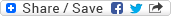## Projection and nested force-gradient methods for quantum field theories

### Dateibereich 6931

1,01 MB in einer Datei, zuletzt geändert am 22.01.2018

DateiDateien geändert amGröße
dc1716.pdf22.01.2018 13:27:131,01 MB

For the Hybrid Monte Carlo algorithm (HMC), often used to study the fundamental quantum field theory of quarks and gluons, quantum chromodynamics (QCD), on the lattice, one is interested in efficient numerical time integration schemes which preserve geometric properties of the flow and are optimal in terms of computational costs per trajectory for a given acceptance rate. High order numerical methods allow the use of larger step sizes, but demand a larger computational effort per step; low order schemes do not require such large computational costs per step, but need more steps per trajectory. So there is a need to balance these opposing effects.

In this work we introduce novel geometric numerical time integrators, namely, projection and nested force-gradient methods in order to improve the efficiency of the HMC algorithm in application to the problems of quantum field theories.

Lesezeichen:
Permalink |Dokumententyp:
Wissenschaftliche Abschlussarbeiten » Dissertation
Fakultäten und Einrichtungen:
Fakultät für Mathematik und Naturwissenschaften » Mathematik und Informatik » Dissertationen
Dewey Dezimal-Klassifikation:
500 Naturwissenschaften und Mathematik » 510 Mathematik
Sprache:
Englisch
Kollektion / Status:
Dissertationen / Dokument veröffentlicht
Dateien geändert am:
22.01.2018
Datum der Promotion:
11.07.2017
Medientyp:
Text